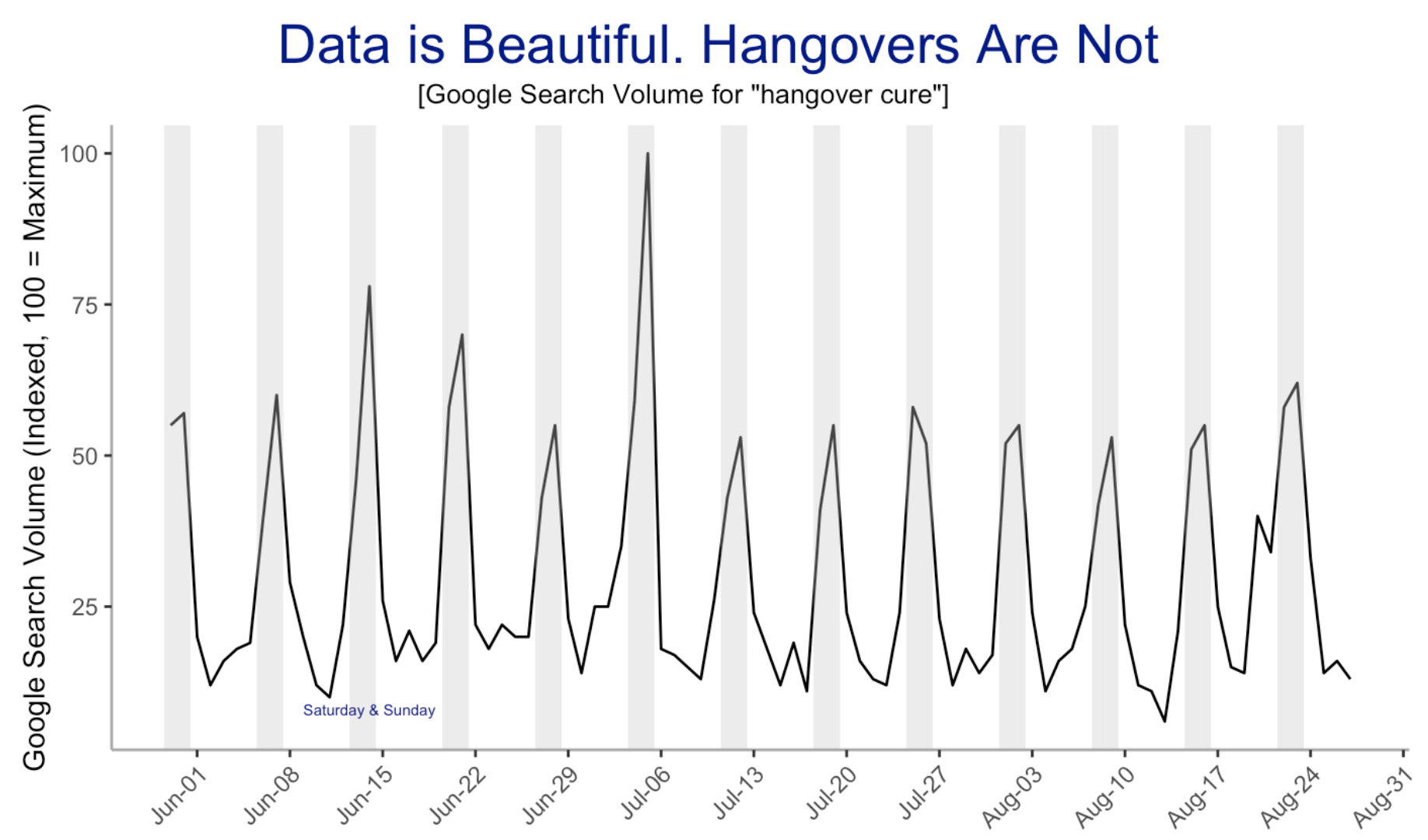# 🍻 💊 Number of People Searching Hangover Cure

This is a remake of chartr.co chart on hangover cure google trend.

When I started working on this mini dataviz project, tidy tuesdays of sort, I was hoping to complete it in a few hours. But as I created the first notebook with Matplotlib, I got greedy and decided to remake the chart using different libraries and languages. The result is this post and a set of notebooks.Remake of chartr.co plot in Altair

# Data

The data comes from Google Trends.

Data Source: Google Trends
Search Term: Hangover Cure
Region: United States (default option)
Date Range: Past 90 days


# Plots

## Python: Matplotlib

The following snippet covers the most of what is need to generate the plot. We pass the dataframe and the function returns the plot.

def generate_plot(df):
fig, ax = plt.subplots(figsize=(14,8))
formatter = mdates.DateFormatter("%b-%d")
ax.xaxis.set_major_formatter(formatter)
# set x-axis rotation
for label in ax.get_xticklabels():
label.set_rotation(40)

ax.plot(df.Day, df.Count, color='black')
ax.bar(df[weekends].Day, *len(df[weekends].Day), alpha=0.2)
ax.set_title("Data is Beautiful: \n Hangovers Are Not", fontsize=30, color="darkblue", pad=30)
ax.set_ylabel("Google Search Volume (Indexed, 100 = Maximum)")

return ax

ax = generate_plot(df)
plt.show()Remake of chartr.co plot in Matplotlib

Matplotlib Notebook

## Python: Altair

In case of Altair, the following snippet will generate the line chart, bar and the annotation. The added benefit of using Altair is that, its extremely easy to create interactive charts. You can also export the chart it the vega format and display it on the web. For example, my crowd density model evaluation post uses this approach. All the plots on that post were generated using Altair.

# Creates the line chart
base = alt.Chart(df).mark_line(color="black").encode(
alt.X('Day:T', axis=alt.Axis(title="", format=("%b %d"), labelAngle=-45, grid=False)),
alt.Y('Count:Q', title="Google Search Volume (Indexed, 100 = Maximum)", axis=alt.Axis(grid=False))
).properties(
title="Data is Beautiful. Hangovers Are Not",
width=1000,
height=500
)

# Create bars
bar = alt.Chart(x_tick_values).mark_bar(size=12, opacity=0.2).encode(
alt.X('Day:T'),
alt.Y('Count:Q')
)

text = (
alt.Chart(df.query("Day == '2020-06-21'"))
.mark_text(dy=280, color="#4F61A1")
.encode(x=alt.X("Day:T"), y=alt.Y("Count:Q"), text=alt.Text("label"))
)

base + bar + text



Altair Notebook

## Julia: Plots

Notice that with very few lines of code, we can create the chart.

# Add the bars
vspan(df.Day, color="#e7e6eb")
plot!(df[:, 1], weekends_df[:, 2], color="red", xticks=(xticks, xlabels), xrotation=25, legend=false, size=(1000,400))
# This fixes the issue of vspan overriding the ylabels
vline!(df.Day, color="#e7e6eb",alpha=0.0, legend=true)
plot!(title="Data is beautiful: \n Hangovers are not", margin=10mm, ylabel="Google Search Volume (Indexed, 100 = Maximum)", yguidefontsize=8)Remake of chartr.co plot in Julia

Julia Notebook

## Julia: Unicode Plot

This library allows us to create the plots in the shell. And while we are not able to replicate the chart here, the output still looks so cool.

function plot(df, plt)
xticks = collect(df.Day:Dates.Day(7):df.Day[lastindex(df.Day)])
if @isdefined plt
plt = lineplot!(plt, df[:, 1], df[:, 2], color=:green)
else
plt = lineplot(df[:, 1], df[:, 2], width=80, title="Data is beautiful. Hangovers are not", ylabel="Google Search Volume (Indexed, 100=Maximum)")
end
# lineplot!(plt, xticks=xticks)
return plt
end

function plot_vspan(plt, df)
weekend_df = weekends(df)
xs = vspan_xs(weekend_df)
ys = vspan_ys(nrow(weekend_df))
for i in 1:2:nrow(xs)-1
lineplot!(plt, xs[i:i+1, 1], ys[i:i+1], color=:yellow)
# println(xs[i:i+1, 1], ys[i:i+1])
end

annotate!(plt, :r, 1, "|| Sat & Sun", color=:yellow)
annotate!(plt, :r, 3, "-- Search Volume", color=:green)

return plt
end


Code

## R: GGPlot2

And to create stunning plots in R, there is ggplot2. It is such a joy to work with, along with all the other tidyverse packages. And this does not mean I did not enjoy working with other packages used above. They’re all fun to work with too.

plt <- ggplot(df, aes(Day, Count)) +
geom_line() +
scale_x_date(date_breaks = "weeks", date_labels = "%b-%d") +
labs(title = "Data is beautiful. Hangovers are not", x="", y="Google Search Volume (Indexed, 100 = Maximum)") +
theme(axis.text.x = element_text(angle=45, hjust=1), panel.grid.major = element_blank(), panel.grid.minor = element_blank(),
panel.background = element_blank(), axis.line = element_line(colour = "darkgray")
)

plt <- plt + geom_vline(aes(xintercept=Day),
data=df %>% filter(weekday %in% c("Saturday", "Sunday")), color="gray",
alpha=0.3, size=2.3)Remake of chartr.co plot in ggplot2

GGPlot Notebook

## Heatmap

Personally, I would prefer to show the data as heatmap.